# GeoGebra applications | Mathematics Centre## GeoGebra applications

Below you will find some sample applications prepared in Geogebra by CNMiKnO PG employees

The application can be started by clicking on "run app on GeogebraTube" or directly on the image below its description.

##### Elementary Functions

Sine and cosine functions (run app on GeoGebraTube).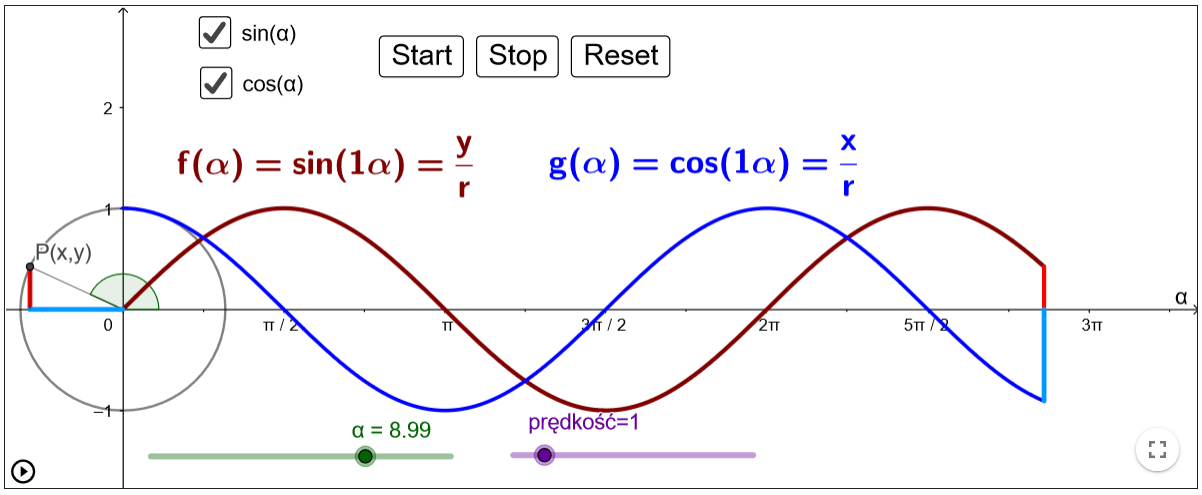##### Number sequences

Limit of a number sequence (run app on GeoGebraTube)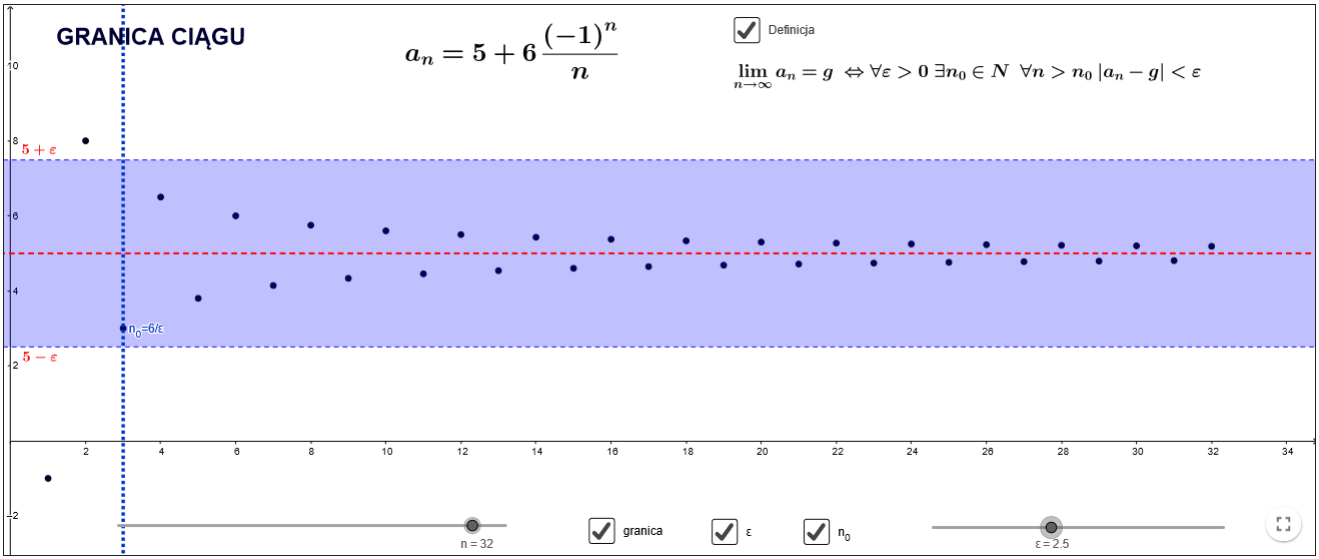##### Limit of a single variable function

Cauchy Definition of limit - examples (run app on GeoGebraTube)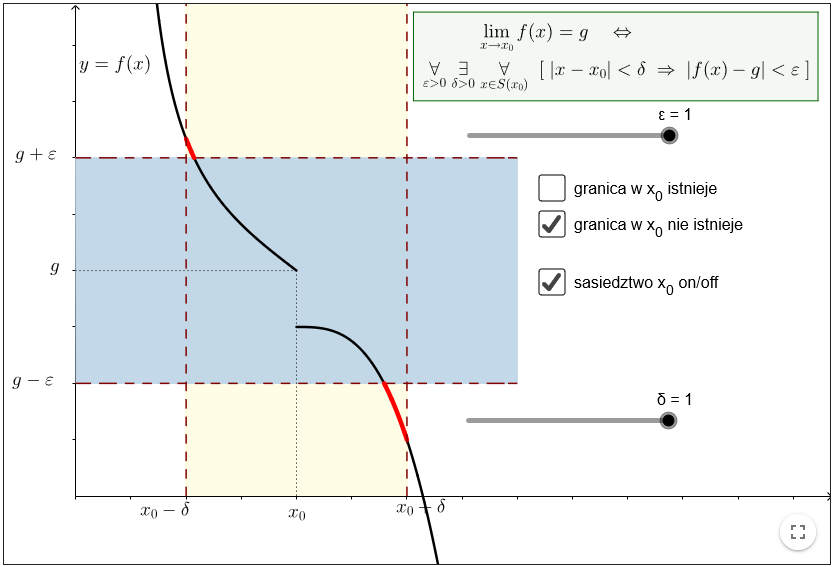##### Derivative of a single variable function

Graphs of functions and their derivatives - examples (run app on GeoGebraTube)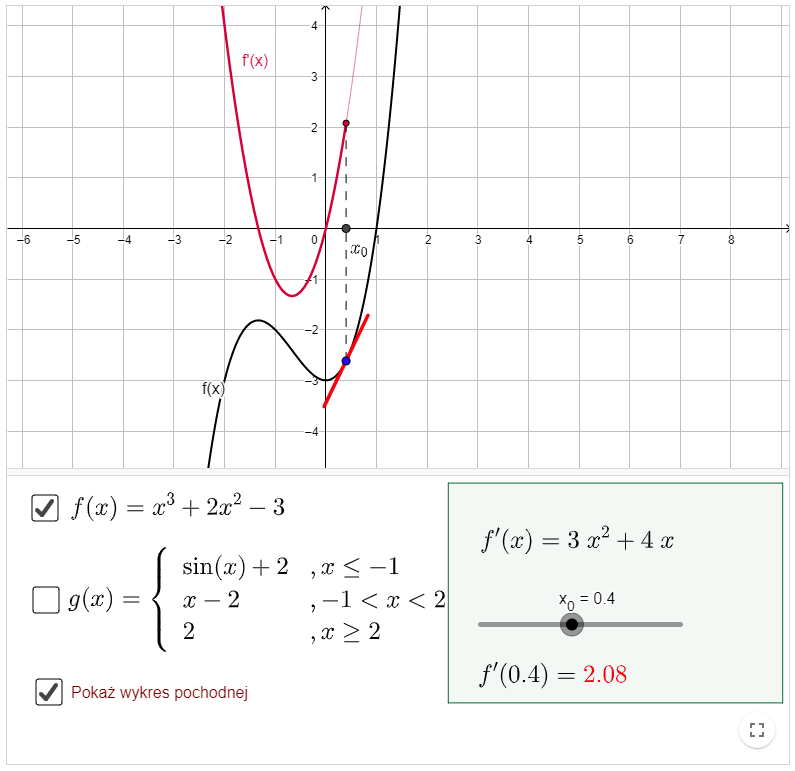Function analysis - example (run app on GeoGebraTube)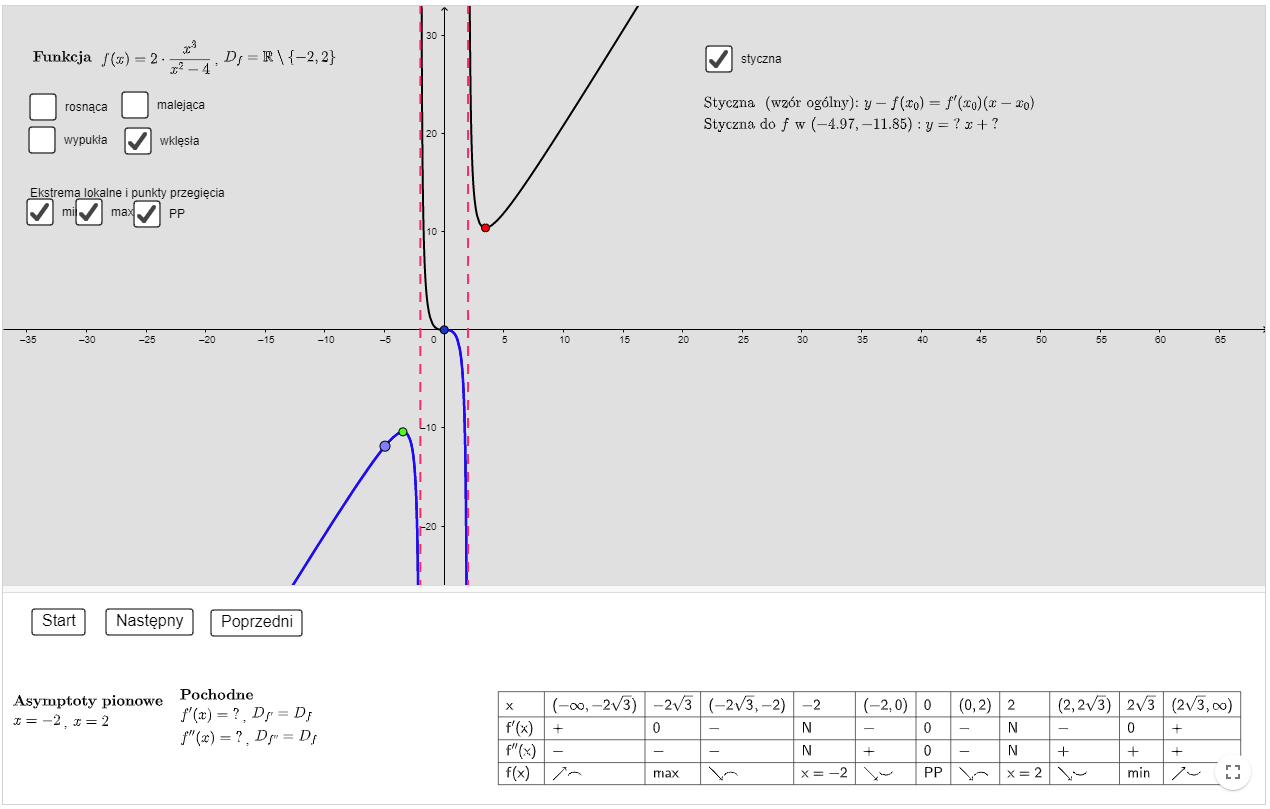##### Improper integral

Improper integral of the first kind - examples (run app on GeoGebraTube)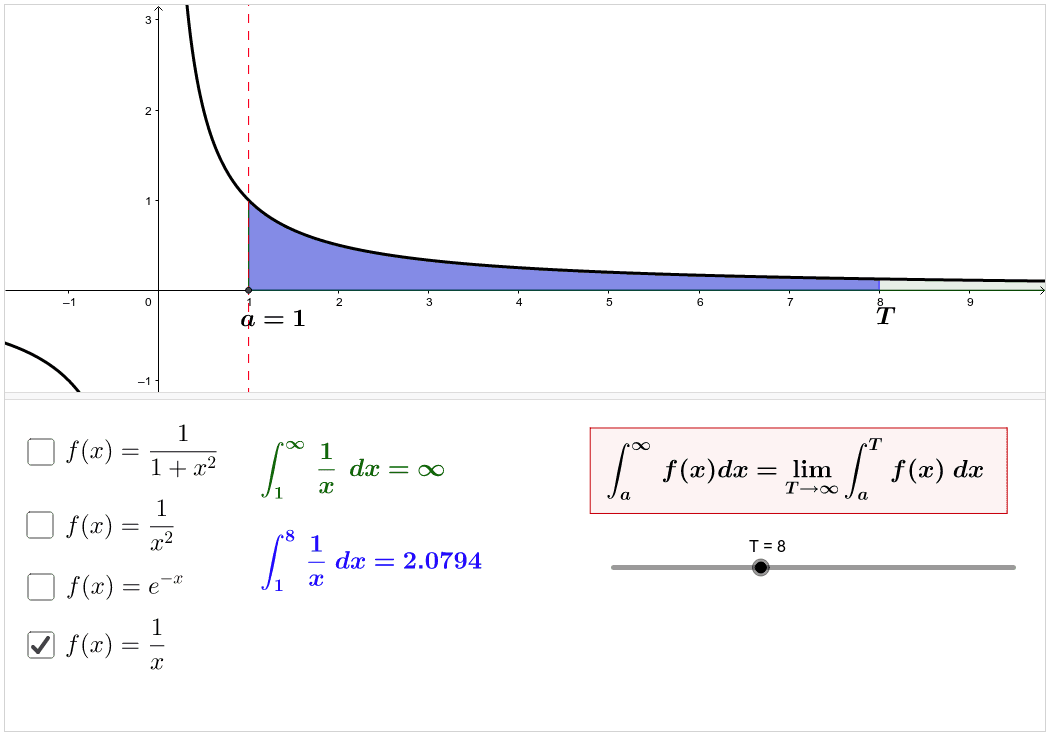Improper integral of the second kind - examples (run app on GeoGebraTube)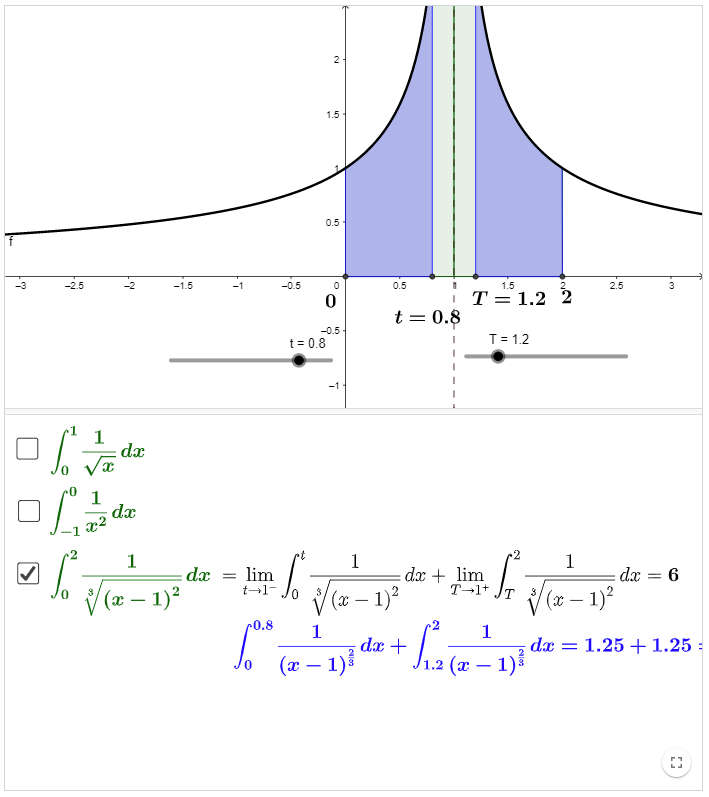##### Implicit functions

Local extreme values of an implicit function - example "Folium of Descartes" (run app on GeoGebraTube)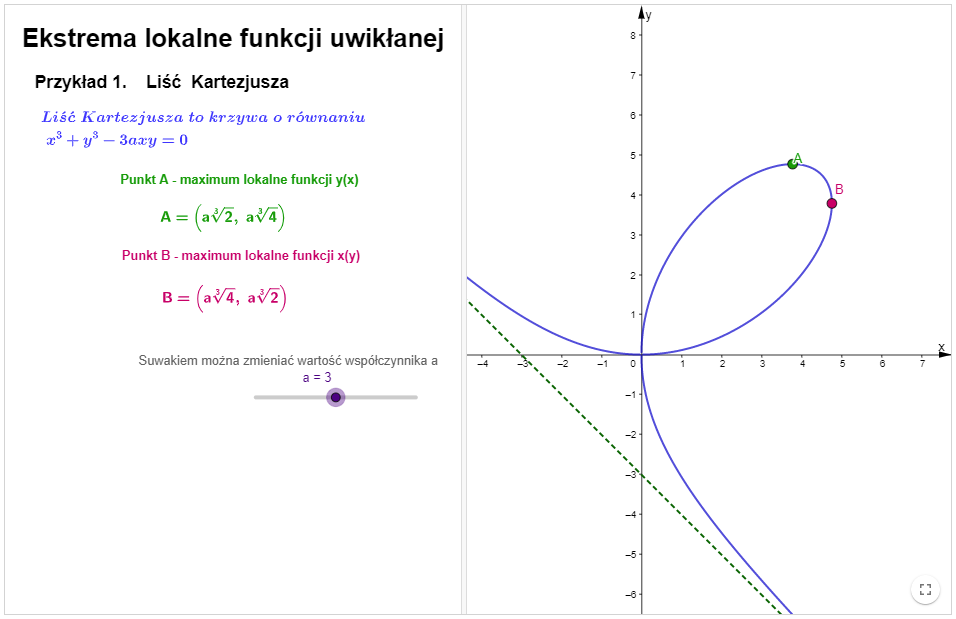Local extreme values of an implicit function - example "Three-leaved rose" (run app on GeoGebraTube)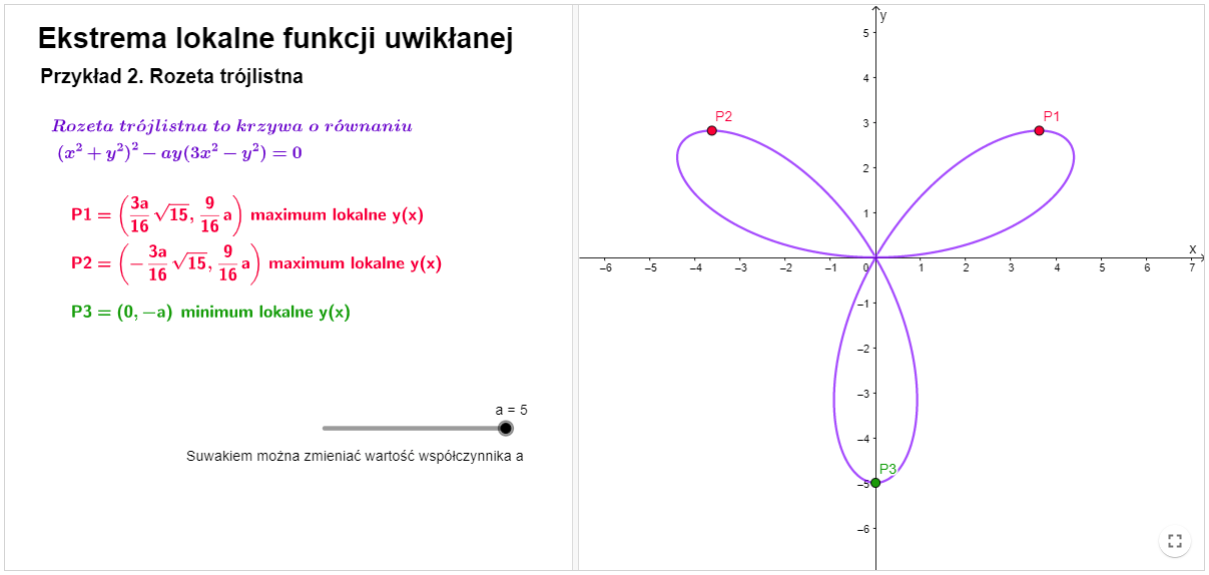##### Change of variables in a tripple integral

Cyllindrical coordinates (run app on GeoGebraTube)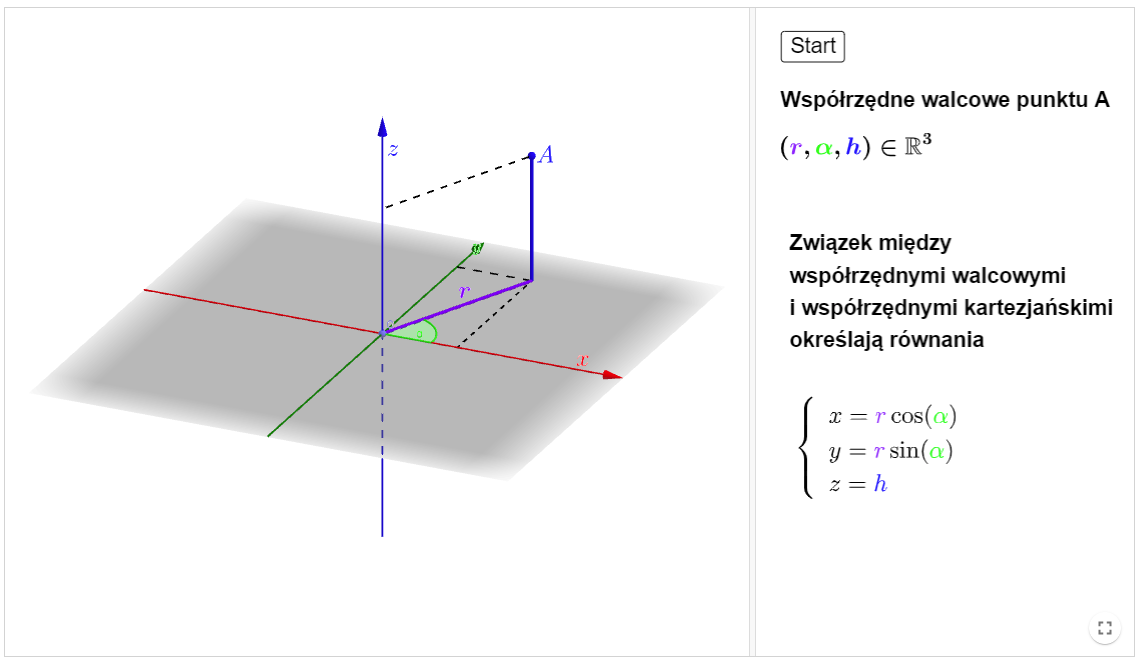Spherical coordinates - type I (run app on GeoGebraTube)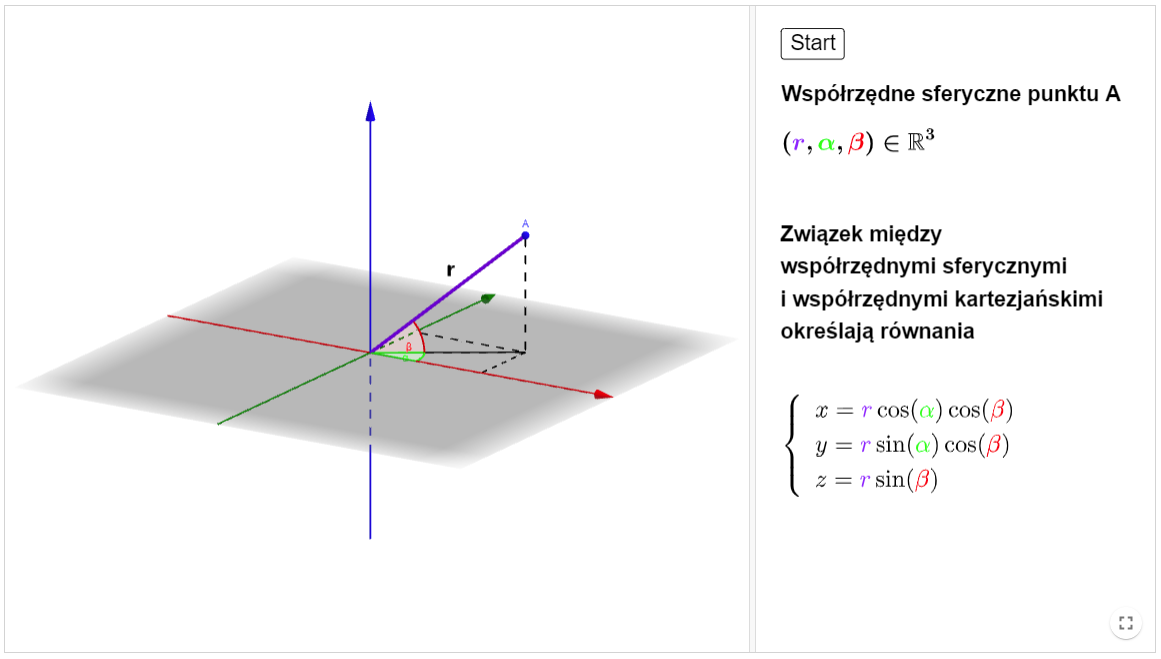##### Number series

Sequence of partial sums - examples (run app on GeoGebraTube)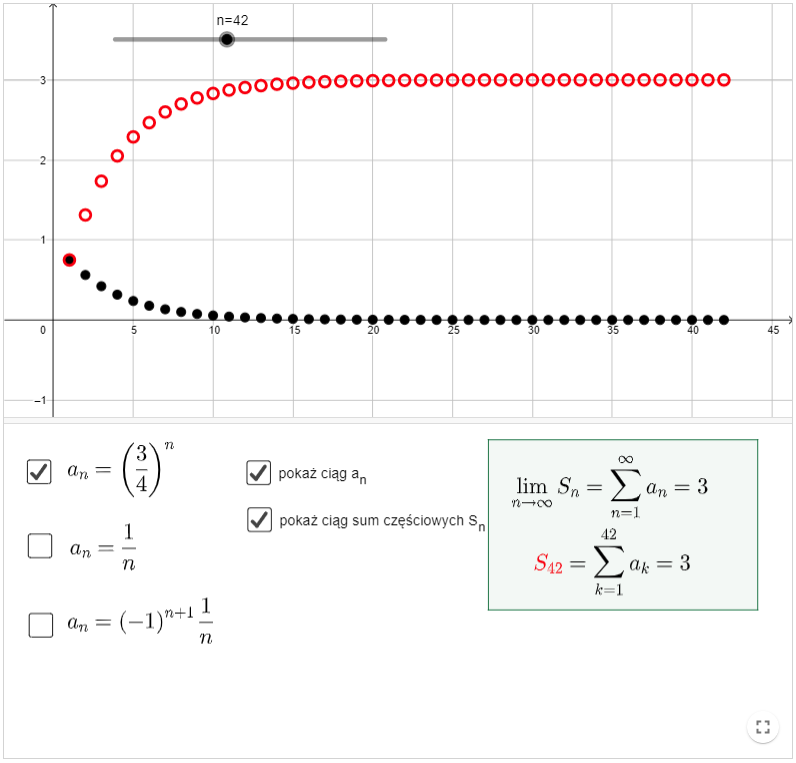##### Fourier Series

Example 1 (run app on GeoGebraTube)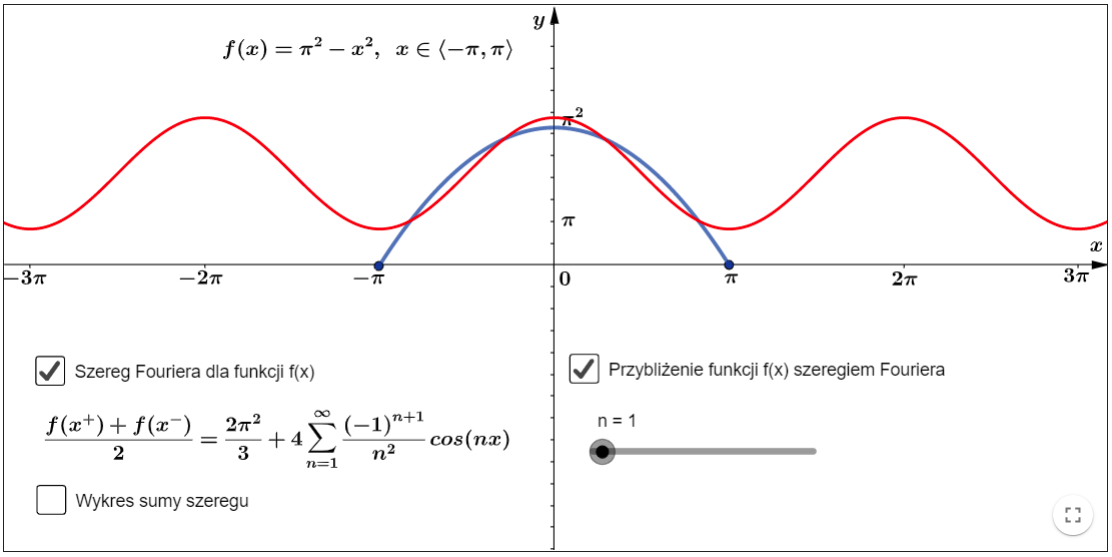Example 2 (run app on GeoGebraTube)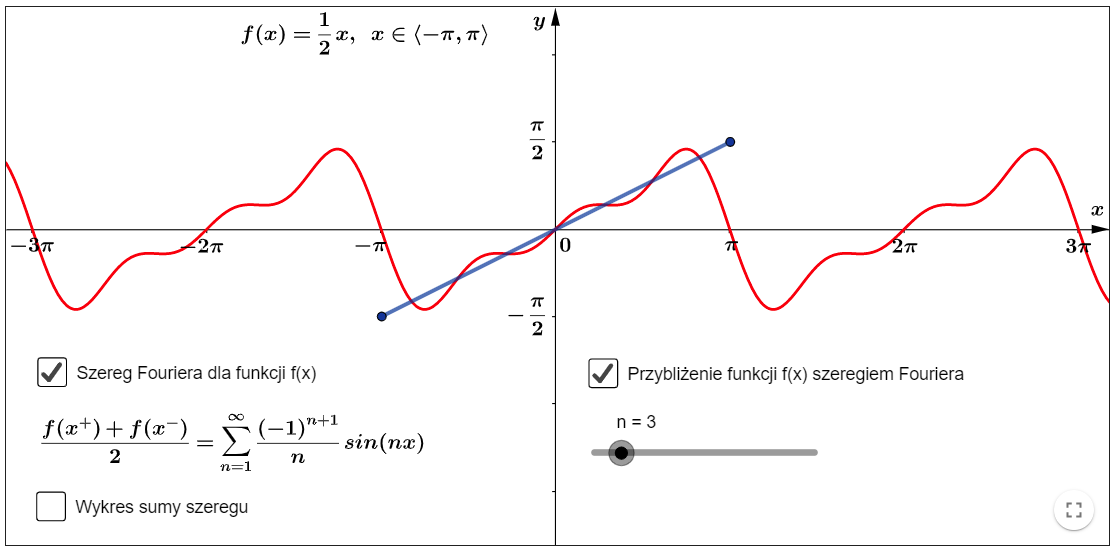##### Complex numbers

Sum of complex numbers (run app on GeoGebraTube)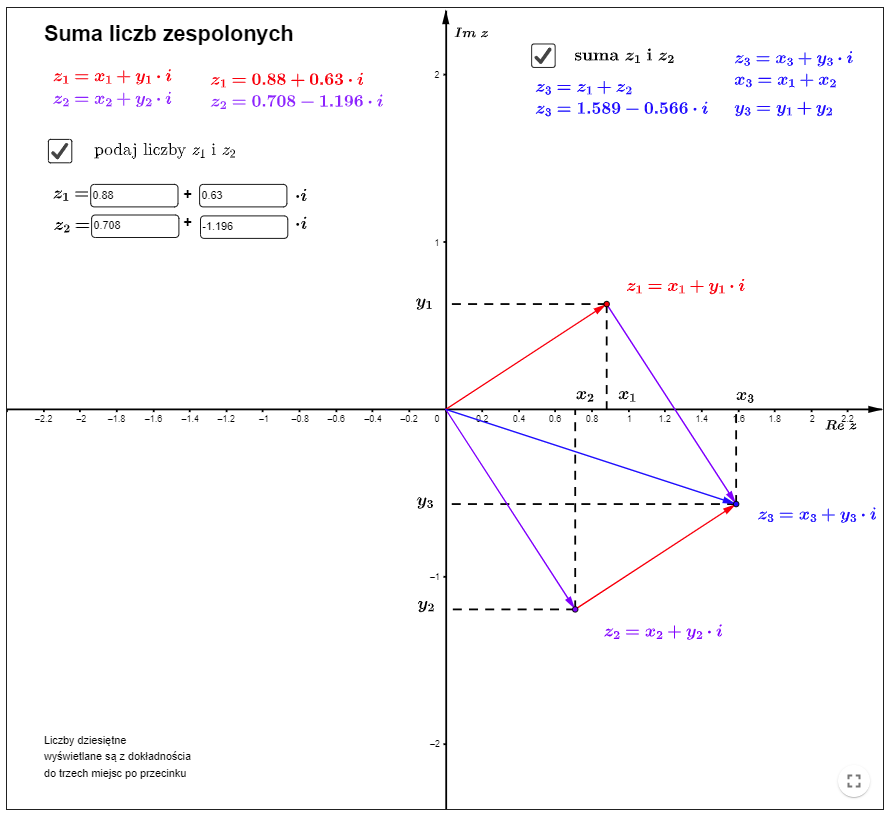Power of a complex number (run app on GeoGebraTube)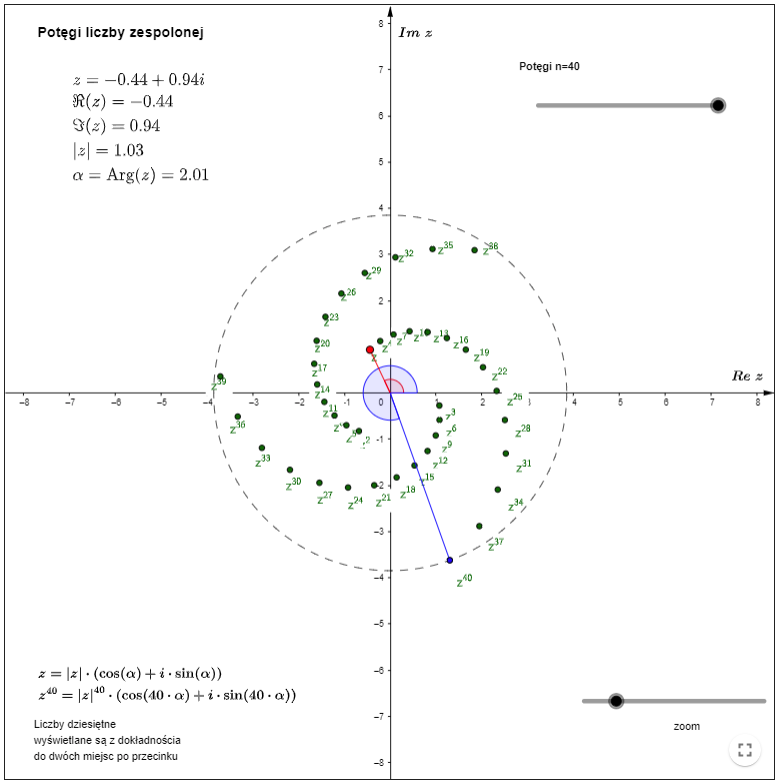Roots of a complex number (run app on GeoGebraTube)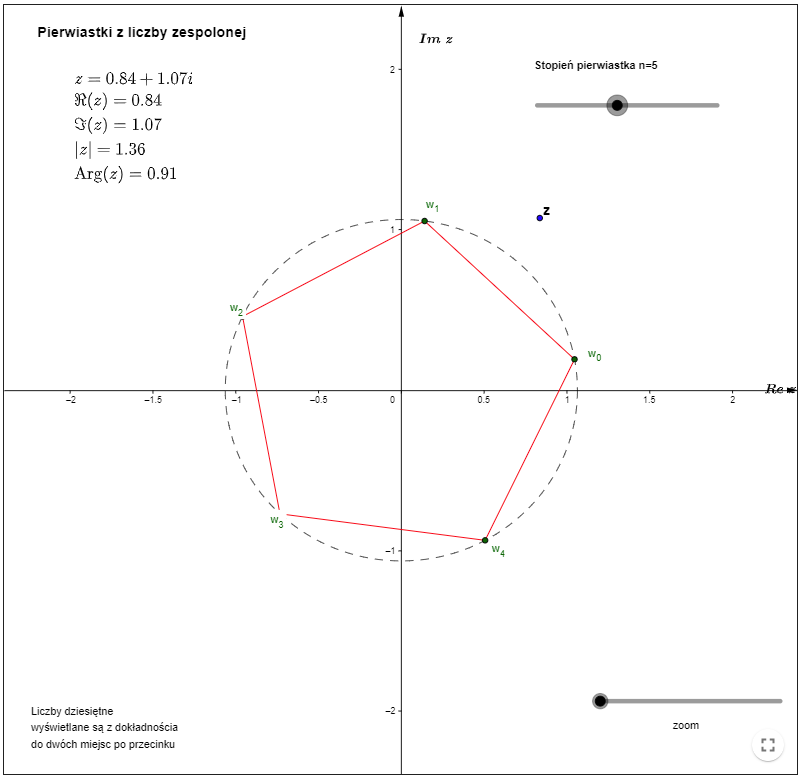Licence CC BY-SA
Mathematics Teaching and Distance Learning Center
Gdańsk University of Technology